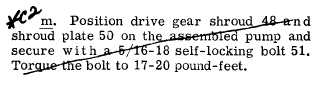Custom Search*C2 PAR   151-153 R E A R    H O U S I N G    R E B U I LD C H A P    5,   SEC  X V I II .   P o s i t i o n    o i l    s e p a r a t o r    p l a t e    a s s e m b ly 36   on   pump   drive   shaft   40   and   retain   it   with two   retaining   rings   35   and   39. .   I n s e r t    k e y    3 4    i n    i t s    g r o o v e    o n    d r i ve shaft   40,   at   the   end   opposite   the   splined   end. Install   drive   gear   33. .   Place   pump   cover   assembly   28   on   the assembly   table,   inside   up,   and   install   the   as- sembled   shaft,   plate   assembly   and   gear   in   the c o v e r . .   Install   the   remaining   separator   plate assembly   23   on   idler   gear   shaft   22   and   retain it   with   two   retaining   rings   35   and   39. .   Position   the   idler   gear   26   over   there- maining   bearing   bore   in   the   cover   and   install the   assembled   idler   gear   shaft   and   plate   as- s e m b l y . .   Install   the   remaining   idler   gear   21   on shaft   22,   and   drive   gear   43   and   key   41   on   the drive   shaft   40. .   I n s t a l l    p u m p    c o v e r    a s s e m b l y    4 4    on p u m p    b o d y    3 2 ,    i n d e x i n g    d o w e l    p i n s    4 6    w i th their    holes. .   Install   the   assembled   cover   assembly 44   and   body   32   over   shafts,   gears   and   sepa- r a t o r    p l a t e s    o n t o    c o v e r    a s s e m b l y    2 8 .    I n s t a ll seven   bolts   27   and   52   to   retain   each   cover   as- s e m b l y .    T o r q u e    t h e m    t o    1 7 - 2 0    p o u n d - f e e t . .   I n s t a l l    b r a k e    c o o l a n t    o i l    p u m p    d r i ve gear   49   on   the   splined   end   of   pump   drive   shaft 40.   Install   lock   pin   42   through   the   gear   and bend   the   ends   of   the   pin   to   lock   it. n .    A s s e m b l e    b o t h    s e t s    o f    b r a k e    c o o l a nt oil   check   valve   components   64   through   68   on brake   coolant   pump   manifold   56. .   Install   pump   assembly   20   and   gasket 54   on   manifold   56   and   secure   with   three   3/8- 16   bolts   60   with   lock   washers   59   and   three 3 / 8 - 1 6    s e l f - l o c k i n g    b o l t s    5 3 .    T o r q u e    t h e    c ap s c r e w s    6 0    t o    2 6 - 3 2    p o u n d - f e e t .    T o r q u e    t i ne self-locking   bolts   53   to   36-43   pound-feet. Section   XVIII. R E A R    H O U S I N G    A S S E M B L Y — R E B U I LD 1 5 2 .    D E S C R I P T I ON Refer   to   pars.   17   and   18   for   the   descrip- tion   of   the   rear   housing   components. 1 5 3 .    D I S A S S E M B L Y    ( f i g s .    3 7 7 ,    3 7 8 ,    3 7 9, 380,   fold-outs   6,   7,   8,   9) a  .   U s i n g    a    9 / 1 6 - i n c h    w r e n c h ,    r e m o v e two self-locking bolts that retain brake air v a l v e    a s s e m b l y    ( f i g .    1 7 5 ) .    R e m o v e    t h e    v a l ve a s s e m b l y. .   Remove   the   retaining   ring   on   the   air valve   assembly   (fig.   175   ). .   R e m o v e    t h e    p i n ,    a n d    d i s a s s e m b l e    t he air   valve   assembly   (fig.   175). .   Using   a   9/16-inch   wrench,   remove   12 cap   screws   and   lock   washers   retaining   brake i n s p e c t i o n     c o v e r s. R e m o v e    t h e    t w o    c o v e rs with   gaskets   (fig.   175). .   Remove   the   retaining   ring   that   retains brake   coolant   pump   idler   gear   and   bearing   on the   idler   gear   shaft   (fig.   176).   Remove   gear and   bearing. .   Remove   the   retaining   ring   that   retains t h e    b e a r i n g    i n    t h e    b r a k e    c o o l a n t    i d l e r    g e ar (fig.   176).   Remove   the   bearing   from   the   gear. 1 2 9